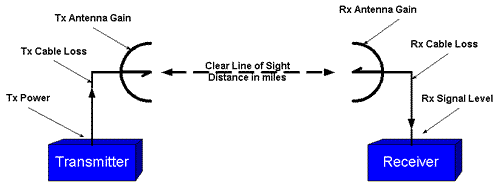CALCULATING SYSTEM OPERATING MARGIN

 SOM Calculation Input Values (fill in all values) Operating Frequency (MHz) Distance (Miles) Tx Power (dBm) Rx Sensitivity (dBm) Tx Cable Loss (dB) Rx Cable Loss (dB) Tx Antenna Gain (dBi) Rx Antenna Gain (dBi)
 Results Rx Signal Level (dBm) Free Space Loss (dB) System Operating Margin (dB)

SOM(dB) = Rx Input Signal Power (dBm) - Rx Sensitivity (dBm)

SOM is a measure in dB of the signal loss your system can with stand before it degrades to the error rate specified at your Receiver Sensitivity threshold. A minimum 20 dB SOM is recommended and many systems are designed with 30 dB+ due to additional circumstances such as inadequate Fresnel clearance, desensitization, intermod etc. This SOM calculator assumes an interference free, free space environment. Note 5W=37 dBm, 1W=30 dBm

Free Space Loss =20Log10(Frequency in MHz) + 20Log10 (Distance in Miles) + 36.6
Rx Signal Level = Tx Power - Tx Cable Loss + Tx Antenna Gain - FSL + Rx Antenna Gain - Rx Cable Loss